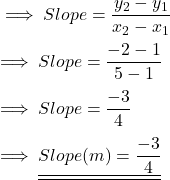## A straight line is drawn through the points A(1,1) and B(5,-2). Calculate the gradient

Question

A straight line is drawn through the points A(1,1) and B(5,-2). Calculate the gradient

in progress 0
2 months 2021-08-02T05:41:23+00:00 1 Answers 3 views 0